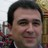/ Matematicas-Compartidas/ Group items tagged Número de OroGroup items tagged

Filter: All | Bookmarks | Topics

Fibonacci Numbers, the Golden section and the Golden String- 1 views

•Fibonacci Numbers and the Golden Section This is the Home page for Dr Ron Knott's multimedia web site on the Fibonacci numbers, the Golden section and the Golden string hosted by the Mathematics Department of the University of Surrey, UK. The Fibonacci numbers are 0, 1, 1, 2, 3, 5, 8, 13, ... (add the last two to get the next) The golden section numbers are ±0·61803 39887... and ±1·61803 39887... The golden string is 1 0 1 1 0 1 0 1 1 0 1 1 0 1 0 1 1 0 1 ... a sequence of 0s and 1s that is closely related to the Fibonacci numbers and the golden section. There is a large amount of information here on the Fibonacci Numbers and related series and the on the Golden section, so if all you want is a quick introduction then the first link takes you to an introductory page on the Fibonacci numbers and where they appear in Nature. The rest of this page is a brief introduction to all the web pages at this site on Fibonacci Numbers the Golden Section and the Golden String together with their many applications.
1 - 1 of 1
Showing 20 items per page